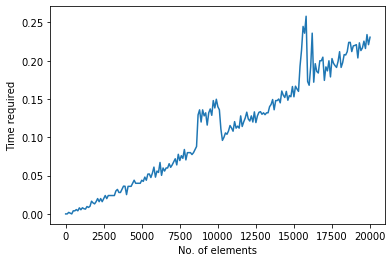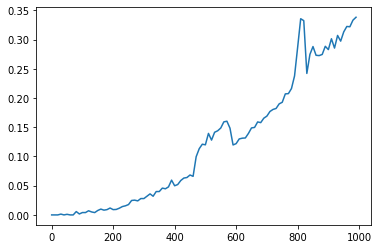# Finding time-complexity of algorithms in Python

Today we’ll be finding time-complexity of algorithms in Python. To do this, we’ll need to find the total time required to complete the required algorithm for different inputs.
The algorithm we’re using is quick-sort, but you can try it with any algorithm you like for finding the time-complexity of algorithms in Python.

Imports:

```import time
from random import randint
from algorithms.sort import quick_sort```

We need the time module to measure how much time passes between the execution of a command.
Next, we use the random module to generate random numbers for our original set of numbers to be sorted.
The algorithms module is imported to get the quicksort code directly. You can also use your own algorithm here. To know more about the algorithms module visit its documentation.

Generating a list of random numbers:

Now that we have imported all our libraries we can start writing our code. We first need an initial array of unsorted elements. For this we use the randint() function. The below code will give us a list of 20001 random integers between 0 to 999.

`list1 = [randint(0,1000) for i in range(20000)]`

## Computing the time required for the algorithm in Python:

We first create an empty list to put all our time values for different inputs.

`times=[]`

Then we run a for-loop, each iteration has a different number of inputs. For each iteration, we first save the time before the execution of the algorithm. Then we run the quicksort algorithm by increasing the number of elements in each iteration. After the algorithm finishes its execution, we save the end time and subtract it with the start time to get the time elapsed. We then append the elapsed time to our list of times.

```for x in range(0,20001,100):
start_time = time.time()
list2 = quick_sort(list1[:x])
elapsed_time = time.time() - start_time
times.append(elapsed_time)```

Let’s see the value of time appended to the list for each iteration.

`print(times)`

Output:

```[0.0, 0.0, 0.0019948482513427734, 0.0009965896606445312, 0.0, 0.004058122634887695, 0.003999948501586914, 0.005641937255859375, 0.004072666168212891, 0.007900714874267578, 0.005433082580566406, 0.008020639419555664, 0.006772041320800781, 0.006285190582275391, 0.00963735580444336, 0.008488178253173828, 0.01000833511352539, 0.0166471004486084, 0.014379501342773438, 0.013109207153320312, 0.01598048210144043, 0.020001888275146484, 0.01599717140197754, 0.019999980926513672, 0.015999794006347656, 0.020000696182250977, 0.023999929428100586, 0.019999265670776367, 0.02401423454284668, 0.023986339569091797, 0.024001359939575195, 0.02399921417236328, 0.023999929428100586, 0.029965639114379883, 0.03199958801269531, 0.027999401092529297, 0.0279998779296875, 0.03200125694274902, 0.03622913360595703, 0.03613924980163574, 0.025216102600097656,
...
...```
`, 0.19364452362060547, 0.19127726554870605, 0.1991591453552246, 0.21184396743774414, 0.19128751754760742, 0.19741511344909668, 0.20798015594482422, 0.20757436752319336, 0.21181511878967285, 0.22397804260253906, 0.2240147590637207, 0.21199965476989746, 0.21940088272094727, 0.2199995517730713, 0.22102975845336914, 0.2036724090576172, 0.22339677810668945, 0.21332645416259766, 0.21673917770385742, 0.225569486618042, 0.21599578857421875, 0.23416709899902344, 0.22098445892333984, 0.2307446002960205]`

We also need the number of inputs at each iteration to plot the graph.

`x=[i for i in range(0,20001,100)]`

## Plotting the graph for finding time complexity

Now, It is time to analyze our findings. Let’s plot our graph with the number of inputs on the x-axis and the time on the y-axis.

```import matplotlib.pyplot as plt
%matplotlib inline
plt.xlabel("No. of elements")
plt.ylabel("Time required")
plt.plot(x,times)```

Output:In the above graph, we can fit a y=xlog(x) curve through the points. So the complexity we computed is O(nlog(n)).

## For worst-case complexity:

Similarly, we can also find the worst-case complexity by passing an already sorted list to the quicksort algorithm.
```list3 = [i for i in range(5000)]
times=[]
for x in range(0,1000,10):
start_time = time.time()
list4 = quick_sort(list3[:x])
elapsed_time = time.time() - start_time
times.append(elapsed_time)
print(times)
x=[i for i in range(0,1000,10)]
```

Output:

```[0.0, 0.0, 0.0, 0.0013897418975830078, 0.0, 0.0010335445404052734, 0.0, 0.0, 0.005716800689697266, 0.001634359359741211, 0.0040531158447265625, 0.0040318965911865234, 0.0070950984954833984, 0.005080223083496094, 0.004001140594482422, 0.007615089416503906, 0.009963035583496094, 0.00817108154296875, 0.009056806564331055, 0.011818647384643555,
...
...```
`, 0.33254265785217285, 0.24218130111694336, 0.2747616767883301, 0.28820180892944336, 0.27323317527770996, 0.27272534370422363, 0.27468228340148926, 0.2886514663696289, 0.2829446792602539, 0.301530122756958, 0.2855985164642334, 0.3072216510772705, 0.29746413230895996, 0.31327223777770996, 0.32255077362060547, 0.32210206985473633, 0.33336806297302246, 0.3383638858795166]`

Let’s plot the graph for the worst-case too.Here, we can clearly see that the degree of the curve is 2. So, the worst-case complexity is O(n^2).